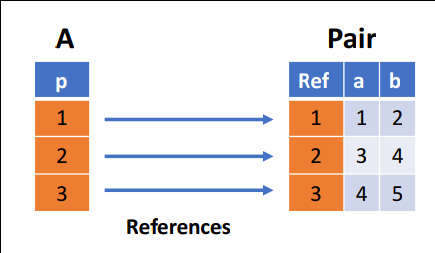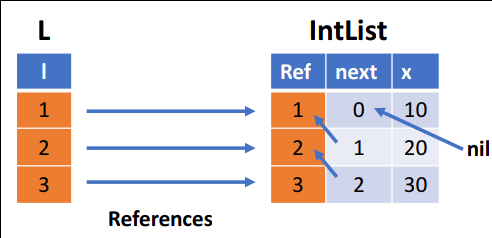Relations store symbol, records, and ADTs using their ordinal numbers. Soufflé uses internal symbol and record tables that conver the ordinal numbers to symbols/records, and vice versa.

## Symbols

Symbols are maintained in the symbol table. A symbol is represented as an ordinal number, and the conversion between its string representation and its ordinal number is handled by the symbol table. The source code of the symbol table can be found here. In relations, the ordinal number is stored. In case, the actual string of a symbol is needed, Soufflé looks up in the symbol table to translate the ordinal number to its string representation. Conversely, if a functor computes a new string or a new string is read by an input directive, the symbol table receives a new entry and an ordinal for this string is computed.

## Records

Records are maintained in the record table. Similar to symbols, a record is stored using its ordinal number, and the conversion between the values of a record and its ordinal number is handled by the record table. The source code of the record table can be found here. Considering the example below,

``````.type Pair = [a: number, b: number]
.decl A(p: Pair)
A([1,2]).
A([3,4]).
A([4,5]).
.output A
``````

generates three new record `[1,2]`,`[3,4]`, and `[4,5]`. Internally, these records are represented and stored as shown in this diagram:For the above example, an `IntList` contains a reference to the next element, which is an `IntList` itself. Internally, this is represented as follows:Abstract Data Type use the record table to convert between their ordinal values and their field values. However, the encoding is more complex since the record table stores records of fixed-length only. Each ADT branch has a unique number in the ADT. The unique number is used in an outer record that refers to the inner record, i.e., `< branch-id, <a1, ...> >`. Using this encoding of ADTs permits the use of fixed-length records.